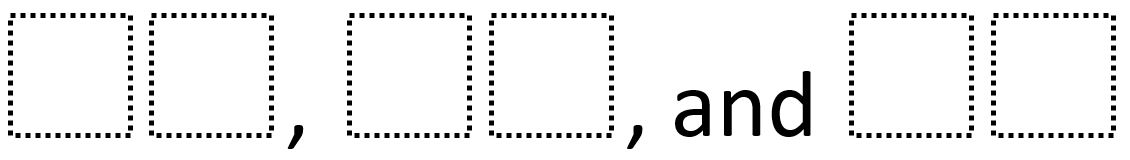Home > Grade 6 > Geometry > Maximizing Rectangular Prism Surface Area

# Maximizing Rectangular Prism Surface Area

Directions: Using the digits 1 through 9 at most one time each, fill in the boxes to list the dimensions of a rectangular prism with the greatest possible surface area.### Hint

Which three digits do you think WON’T be included in the dimensions? Why not? (from Julie Wright

### Answer

94 x 85 x 76 (from Julie Wright)

Source: Robert Kaplinsky

## Converting a Fraction to a Decimal

Directions: Using the digits 1 to 9, at most one time each, place a digit …

### 2 comments

1.If it’s ab x cd x ef [ab is really 10a + b, etc.], then SA = 2[ab x cd + ab x ef + cd x ef]. Maximizing this is the same as maximizing 100[a x c + a x e + c x e] + 10 [a x d + b x c + a x f + b x e + c x f + d x e] + 1 [b x d + b x f + d x f]. So a, c, and e ought to be 7, 8, 9 in arbitrary order (since they’re the ones whose products are multiplied by 100) and b, d, f ought to be 4, 5, 6 in some order (clearly bigger results than you’d get with 1, 2 or 3). The last term [with 1] doesn’t depend on the order of 4, 5, 6, but the middle term [with 10 coefficient] does. If a=9, c=8, e=7, then to maximize [rearranged] (c + e) x b + (a + e) x d + (a + c) x f, f should be biggest (because a + c is the biggest sum) and b should be smallest (like c + e).

So I think the answer is 94 x 85 x 76.

Possible hint: Which three digits do you think WON’T be included in the dimensions? Why not?

•Thanks Julie! I added your info to the problem.